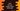# How to remove empty values while split in Java## How to remove empty values while split in Java:

Suppose a string is given, words are separated by a separator, including empty values. We need to remove the empty values and put the words in an array.

Suppose,  the string is:

``"one,two,,three,,four,,, ,"``

We have empty words in this string, where words are separated by comma.

Now, let’s see how to get the non-empty words in a separate array in different ways:

### By using stream:

We can :

• Convert the string to an array of words by using split
• Convert that to a stream
• Remove all empty values using filter
• Convert it back to an array by using toArray

Below is the complete program:

``````import java.util.Arrays;

public class Main {
public static void main(String[] args) {
String givenString = "one,two,,three,,four,,    , ,";

String[] resultArray = Arrays.stream(givenString.split(",")).filter(e -> e.trim().length() > 0).toArray(String[]::new);

System.out.println(Arrays.toString(resultArray));
}
}``````

It will work. It will give the below result:

``[one, two, three, four]``